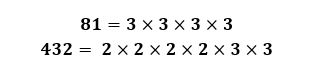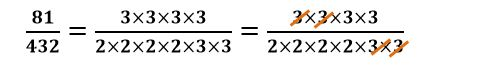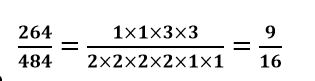# How to cancel fractions using prime factors

Want to find out how to cancel fractions using prime? We've provided a step-by-step guide of how to cancel fractions using prime factors to teach you everything you need to know.## Step 1: Express the numerator and denominator of a fraction as product of its prime factors

If 72/80 is to be simplified, we express 72and 80 as products of their prime factors.

## Step 2: Cancel out common prime factors

Cancel out common prime factors shared by the numerator and the denominator.

## Step 3: Multiply the remaining prime factors

After cancelling out all of the common prime factors, multiply the remaining prime factors to find the simplified fraction.

## Examples of how to cancel fractions using prime factors

Q1) Cancel out common prime factors from 81/432 to simplify the fraction.

We have 81 and 432 as product of their prime factors.Cancel the common prime factors shared by the numerator and denominator.Once we’ve cancelled the common prime factors shared between 81 and 432, multiplying the remaining prime factors.That’s why we have 9/16 as the lowest terms of the fraction.

#### Join today

The fastest way to practice

Unlock our complete testing platform and improve faster that ever.